# Acceleration: changing velocity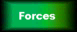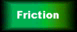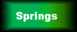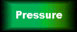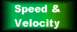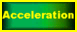Acceleration is how much your velocity changes each second

Velocity is measured in metres per second (m/s),
so acceleration is measured in metres per second per second (written as m/s/s or m/s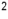)

No, it's not a misprint, it's really called that.
It's how much your velocity (metres per second), changes each second (per second).

We work out acceleration using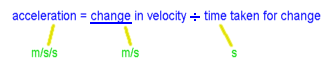Example: a car accelerates from 8 m/s to 20 m/s, and takes 6 seconds to do it.                       What is the acceleration? Answer: change in velocity is 20 minus 8 = 12 m/s. Time taken for change is 6 seconds.                                  Acceleration = 12 divided by 6 = 2 m/s/s (that's a pretty impressive car!)

Force and acceleration:

If the forces on an object are in balance, then its velocity will be constant (see Forces page)

If the forces aren't in balance, then the object will accelerate - which may mean speeding up, slowing down, or changing direction.

 If we apply an unbalanced force to an object, it will accelerate. If we apply twice the force, we'd expect to get twice the acceleration. If we apply the same force to an object with twice the mass, we'd expect to get only half the acceleration.

Need to work out how much it accelerates? See the bottom of this page

We have more flexibility to cope with the calculations if we use an equation: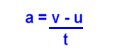Where a = acceleration (m/s/s)
v = final velocity (m/s)
u = initial (starting) velocity (m/s)
t = time (seconds)

Thus v - u is the change in velocity

 Example: a wombat falls out of a tree into a vat of custard. It accelerates at 10 m/s/s, and falls for 5 seconds. How fast is it going when it hits the custard? Answer: first, realise that the wombat starts from rest, so u = 0.              we have: a = 10 m/s/s, t = 5 sec, and we want v.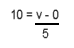, so v = 10 x 5 = 50 m/s                                                            (that's just over 111 miles per hour. Wombat puree)

Newton's Second Law tells us how much an object accelerates if the forces are unbalanced. It comes down to an equation:

F = ma

where F = force (in Newtons)
m = mass of object (in kilogram)
a = acceleration (in metres per second per second)

 Example: a guinea pig of mass 1 kg sits on a skateboard of mass 2kg. If the skateboard is pushed, and accelerates at 4 m/s/s, how big is the force pushing it? Answer: first, we need to add the mass of the guinea pig and the skateboard together.               That's 1kg + 2kg = 3kg                we know that acceleration = 4 m/s/s using F = ma, we have F = 3 x 4 = 12 Newtons (hope the guinea pig was holding on tight)